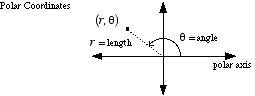index: click on a letter A B C D E F G H I J K L M N O P Q R S T U V W X Y Z A to Z index index: subject areas numbers & symbols sets, logic, proofs geometry algebra trigonometry advanced algebra & pre-calculus calculus advanced topics probability & statistics real world applications multimedia entrieswww.mathwords.com about mathwords website feedback

 Polar Coordinates A way to describe the location of a point on a plane. A point is given coordinates (r, θ). r is the distance from the point to the origin. θ is the angle measured counterclockwise from the polar axis to the segment connecting the point to the origin. Note: With polar coordinates a given point has many possible representations. θ has many possible values depending on which coterminal angle is chosen, and r can be positive or negative.See also